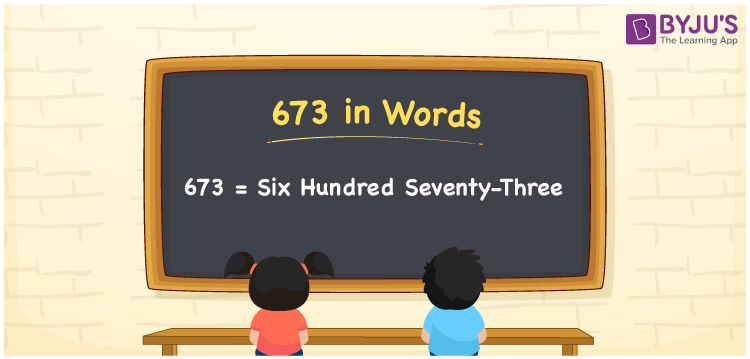# 673 in Words

Six hundred seventy-three is the number name of 673. In Mathematics, the number 673 is considered to be a cardinal number. For transforming the number 673 into words, we use the place value system. For example, the cost of 4 kg oranges is Rs. 673. It can also be written as “The cost of four kg oranges is Rs. Six hundred seventy-three. Now, let us discuss the procedure of writing the number 673 in words in detail.

 673 in Words: Six Hundred Seventy-three. Six Hundred Seventy-three in Numerical Form: 673.

## 673 in English Words## How to Write 673 in Words?

The following table depicts the place values of 673.

 Hundreds Tens Ones 6 7 3

The expanded form of 673 is as follows:

= 6 × Hundred + 7 × Ten + 3 × One

= 6 × 100 + 7 × 10 + 3 × 1

= 600 + 70 + 3

= 673

= Six hundred seventy-three

Hence, 673 in words is six hundred seventy-three.

The number 673 appears after 672 and before 674.

673 in words – Six hundred seventy-three

Is 673 an odd number? – Yes

Is 673 an even number? – No

Is 673 a perfect square number? – No

Is 673 a perfect cube number? – No

Is 673 a prime number? – Yes

Is 673 a composite number? – No

## Frequently Asked Questions on 673 in Words

Q1

### Write 673 in words.

673 in words is six hundred seventy-three.

Q2

### Simplify 600 + 73, and express it in words.

Simplifying 600 + 73, we get 673. Hence, 673 in words is six hundred seventy-three.

Q3

### Is 673 a prime number?

Yes, 673 is a prime number.